## Panini stickers, birthdays and a collision problem

Written on 29 January 2014, 05:16pm

Tagged with: , , ,

### 1. Some princesses

Recently I bought a Panini sticker album with Frozen. I know, but I like animated movies and it reminded me of the time when I was collecting and trading stickers myself 🙂

Some notes: In theory, you should not have any duplicate in a pack of 5 stickers.
Also, all the 192 stickers have the same representation in the envelopes. From my past experience, I know that’s not true. Even after swapping stickers with friends, some of the stickers were impossible to find.
Prices are pack of 5 stickers – 0.6€, 50 stickers 6€, 250 stickers – 30€.

### 2. The Birthday problem

So, I wanted to play a bit with the probabilities involved in this little collection game. The starting point was the Birthday problem, and the apparent paradox that in a class of 23 students, the probability of having 2 students with the same birthday is 50%.
The formula to find this probability is: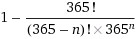The graphical representation of this formula is below (you can also plot it on Wolfram|Alpha):

### 3. The Collision problem

Moving on; the birthday problem is a particular case of the collision problem. Next I will be playing with `n`, `d` and `p`:
```n random numbers from range [1,d] p = probability that two numbers are the same```
There are 2 associated formulas:
1. n and d given, find out p (what is the probability that at least two numbers are the same; or in stickers slang, the duplicates probability)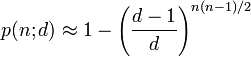2. p and d given, find out n (how many numbers we should have in the interval [1,d] in order to meet the probability p)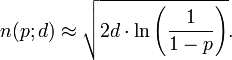Back to the sticker album 🙂
Using the first formula plus Wolfram|Alpha, we get the following figures: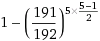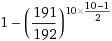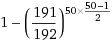``````  n   d   p
5 192     5%
10 192   20%
17 192   50%
50 192  99.8%
192 192  100%
``````

### 4. The Collectors problem

The Collectors problem provides an answer to the question ‘how many stickers are necessary in order to collect all the 192?’ (stickers swapping ignored).
The long answer: there is a formula to solve the collectors problem. It reflects how the probability of collecting a distinct sticker decreases with every new sticker collected:

`````` x defined as number of stickers necessary to collect all the 192 distinct ones
x = 192/192 + 192/191 + 192/190 + .... + 192/1
x = 192 * (1/192 + 1/191 + ... + 1/1)
x = 192 * sum (1/i), i=1 to n
x = 1120.76``````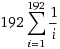Wolfram|Alpha formula

The formula above helps also if you have a number of stickers and you want how many of them are distinct:

``````x = total number of stickers
x/192 = sum (1/i), i=k to 192
y = the projected number of distinct stickers from the batch of x stickers
y = 192 - k

x    y
192   120
250   139
500   177
650   186
832   190
928   191
1121    192
``````

### Conclusions

1. In a class of 23 students, there are 50% chances that two of them have the same birthday
2. In a Panini pack of 17 stickers (out of 192 possible stickers), there are 50% chances to have a duplicate
3. If there are 192 possible stickers, you would need to collect at least 1121 stickers in order to complete the album
4. Buying 192 stickers (out of 192 possible stickers) will probably leave you with about 120 distinct stickers (rest of them being duplicates).

This is a quite theoretical approach. For a more practical one, including a strategy for the passionate collectors, see the paper below:

Abstract:
We consider some issues related to the famous Panini stickers devoted to the football world cup. In particular, we address the following questions: is there a planned shortage of some stickers? What is a good cost-effective strategy to fill in an album?